# Plot mean and standard deviation using ggplot2 in R

• Difficulty Level : Hard
• Last Updated : 21 Jul, 2021

An error bar shows the confidence and precision in a set of measurements or calculated values based on the errors that occur in the data set. It helps visually display the errors in an area of the data frame and shows an actual and exact missing part. As a descriptive behavior, error bars provide details about variances in data as well as recommendations to make changes so that data becomes more insightful and impactful for users.

## Getting Started

geom_errorbar(): This function is used to produce the error bars.

Syntax:

geom_errorbar(mapping = NULL, data = NULL, stat = “identity”, position = “identity”, …)

Example: Plot to display mean and standard deviation on a barplot.

## R

 `df<-``data.frame``(Mean=``c``(0.24,0.25,0.37,0.643,0.54),``               ``sd=``c``(0.00362,0.281,0.3068,0.2432,0.322),``               ``Quality=``as.factor``(``c``(``"good"``,``"bad"``,``"good"``,``                                   ``"very good"``,``"very good"``)), ``               ``Category=``c``(``"A"``,``"B"``,``"C"``,``"D"``,``"E"``),``               ``Insert= ``c``(0.0, 0.1, 0.3, 0.5, 1.0))`` ` `# Load ggplot2``library``(ggplot2)`` ` `ggplot``(df, ``aes``(x=Category, y=Mean, fill=Quality)) +``  ``geom_bar``(position=``position_dodge``(), stat=``"identity"``,``           ``colour=``'black'``) +``  ``geom_errorbar``(``aes``(ymin=Mean-sd, ymax=Mean+sd), width=.2)`

Output: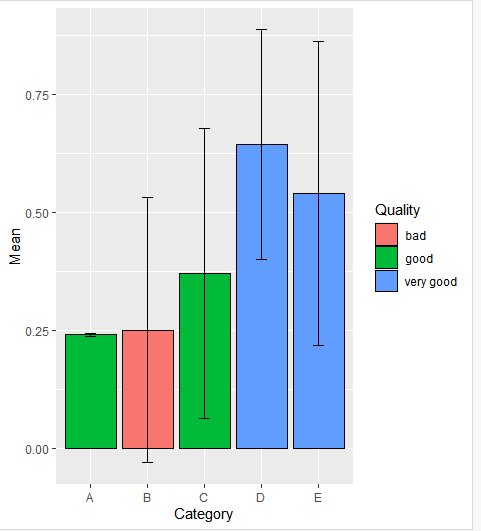Now let us look at the point plot, if we want to add points to the same dataframe, simply add geom_point().

Syntax:

geom_point(mapping = NULL, data = NULL, stat = “identity”, position = “identity”,…, na.rm = FALSE,show.legend = NA,inherit.aes = TRUE)

Example1: Plot with points

## R

 `# creating a data frame df``df<-``data.frame``(Mean=``c``(0.24,0.25,0.37,0.643,0.54),``               ``sd=``c``(0.00362,0.281,0.3068,0.2432,0.322),``               ``Quality=``as.factor``(``c``(``"good"``,``"bad"``,``"good"``,``                                   ``"very good"``,``"very good"``)), ``               ``Category=``c``(``"A"``,``"B"``,``"C"``,``"D"``,``"E"``),``               ``Insert= ``c``(0.0, 0.1, 0.3, 0.5, 1.0))`` ` `# plot the point plot``p<-``ggplot``(df, ``aes``(x=Category, y=Mean, fill=Quality)) + ``  ``geom_point``()+``  ``geom_errorbar``(``aes``(ymin=Mean-sd, ymax=Mean+sd), width=.2,``                ``position=``position_dodge``(0.05))`` ` `p`

Output: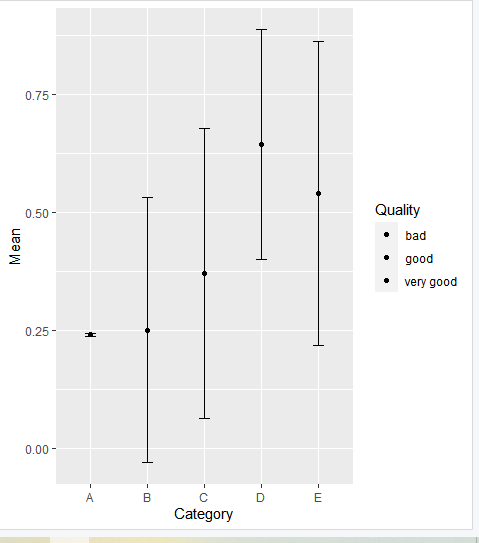Different methods are used by different groups to illustrate their differences. Alternatively, dot plots or point plots are used. To tell ggplot that a column or dot represents a mean, we need to indicate a mean statistic. Let us explore this in detail using a different dataframe. To do this, we can use ggplot’s “stat”-functions.

Let’s visualize the results using bar charts of means. In place of using the *stat=count>’, we will tell the stat we would like a summary measure, namely the mean. Then, the dataframe is divided into groups, and the mean and standard deviation for each is noted and plotted. This can be done using summarize and group_by().

File in use: Crop_recommendation

Example: Plot with mean and standard deviation for each group.

## R

 `# load crop_recomendation csv file and ``# store it in ds``ds <- ``read.csv``(``"Crop_recommendation.csv"``, header = ``TRUE``)`` ` `ggplot``(ds, ``aes``(x=label, y=temperature)) + ``geom_boxplot``() `` ` `# create a new dataframe crop_means``crop_means <- ds %>% ``  ``group_by``(label) %>% ``  ``summarize``(mean_temperature=``mean``(temperature)) ``crop_means`` ` `# Creating barplots of means``ggplot``(crop_means, ``aes``(x=label, y=mean_temperature)) +``geom_bar``(stat=``"identity"``) `

Output: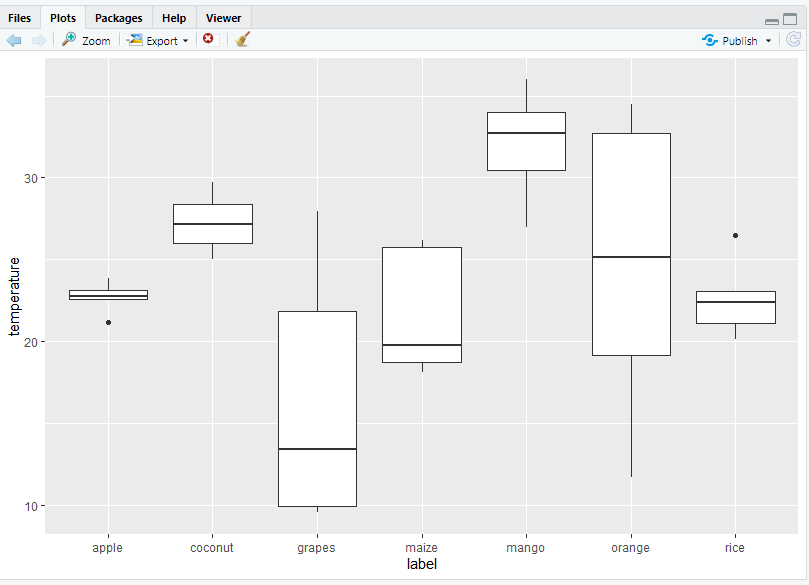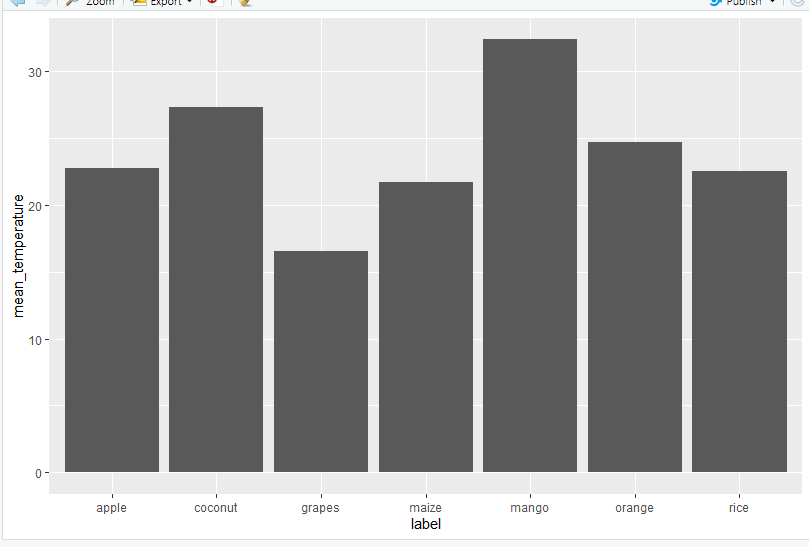Now, if you want to point the point plot then you can also do that by using the geom_point() function.

Syntax:

geom_point(stat=”summary”, fun.y=”mean”)

Example: point plot

## R

 `# load crop_recomendation csv file and ``# store it in ds``ds <- ``read.csv``(``"Crop_recommendation.csv"``, header = ``TRUE``)`` ` `ggplot``(ds, ``aes``(x=label, y=temperature)) + ``geom_boxplot``() `` ` `# create a new dataframe crop_means``crop_means <- ds %>% ``  ``group_by``(label) %>% ``  ``summarize``(mean_temperature=``mean``(temperature)) ``crop_means`` ` `# creating point plots of means``ggplot``(ds, ``aes``(x=label, y=temperature)) + ``geom_point``(stat=``"summary"``, fun.y=``"mean"``) `

Output: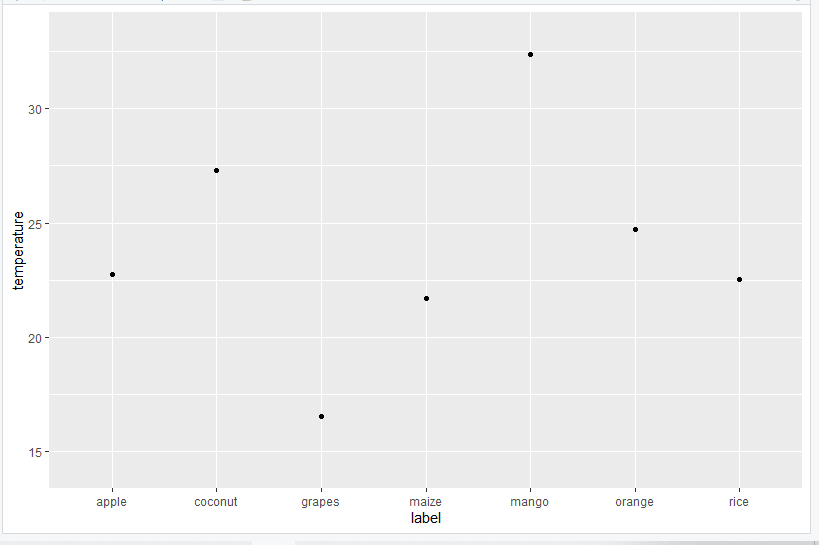For plotting Standard Deviation(SD) you need to use geom_errorbar(). First, we can create a new dataset, which is the most labor-intensive way of creating error bars. We will also calculate the standard error this time (which equals the standard deviation divided by the square root of N).

Syntax:

geom_errorbar()

Parameters:

• ymin or xmin : Lower Value of custom point
• ymax or xmax: Upper Value of custom point
• height: height of errorbar
• alpha: Opacity of error bar
• color: Color of error bar
• group: Differentiate points by group
• linetype
• size

Example: Plotting standard deviation

## R

 `# load a crop recommendation csv file dataset``ds <- ``read.csv``(``"Crop_recommendation.csv"``, header = ``TRUE``)`` ` `# create a new dataframe crop_means_Se``crop_means_se <- ds %>%  ``  ``group_by``(label) %>% ``  ``summarize``(mean_N=``mean``(N), ``            ``sd_N=``sd``(N), ``            ``N_N=``n``(), ``            ``se=sd_N/``sqrt``(N_N), ``            ``upper_limit=mean_N+se, ``            ``lower_limit=mean_N-se ``  ``) `` ` `crop_means_se`` ` `ggplot``(crop_means_se, ``aes``(x=label, y=mean_N)) + ``geom_bar``(stat=``"identity"``) + ``geom_errorbar``(``aes``(ymin=lower_limit, ymax=upper_limit))`

Output: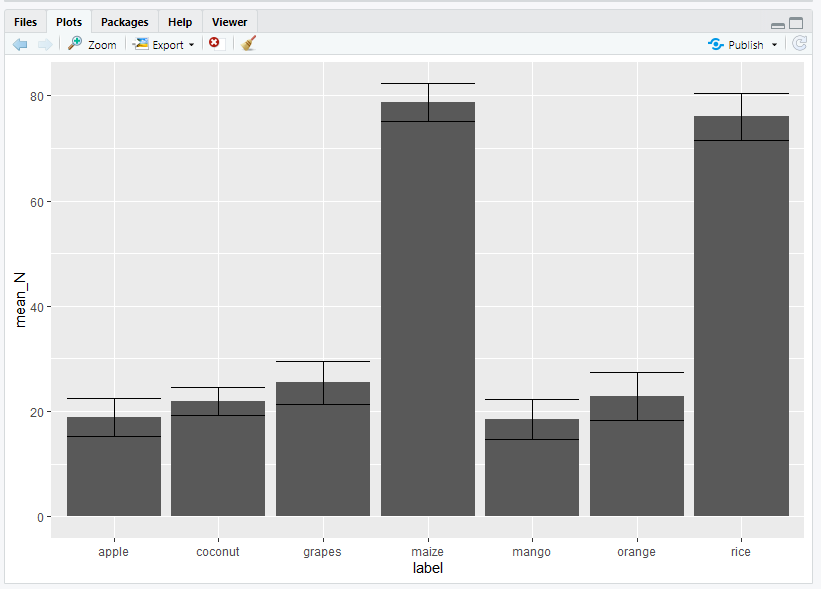You can also create your own “se” function by using geom_errorbar(). Xmin & Xmax and Ymin & Ymax can be used to plot the errorbar horizontally or vertically.

Syntax:

geom_errorbar(stat=”summary”,fun.ymin=function(x){mean(x-sd(x)/sqrt(length(x))}, fun.ymax=function(x){mean(x)+sd(x)/sqrt(length(x))}).

Here, we calculate ymin and ymax values to plot the errorbar vertically, and these values are created by a separate function in which average of( x-sd(x)/sqrt(length(x)) is calculated for a minimum of y or ymin and the average of (x+sd(x)/sqrt(length(x)) is calculated for a maximum of y or ymax.

Example: Plotting standard deviation

## R

 `# load a crop recommendation csv file dataset``ds <- ``read.csv``(``"Crop_recommendation.csv"``, header = ``TRUE``)`` ` `ggplot``(ds, ``aes``(x=label, y=N)) + ``geom_bar``(stat=``"summary"``, fun.y=``"mean"``) + ``  ``geom_errorbar``(stat=``"summary"``, ``                ``fun.ymin=``function``(x) {``mean``(x)-``sd``(x)/``sqrt``(``length``(x))}, ``                ``fun.ymax=``function``(x) {``mean``(x)+``sd``(x)/``sqrt``(``length``(x))})`

Output: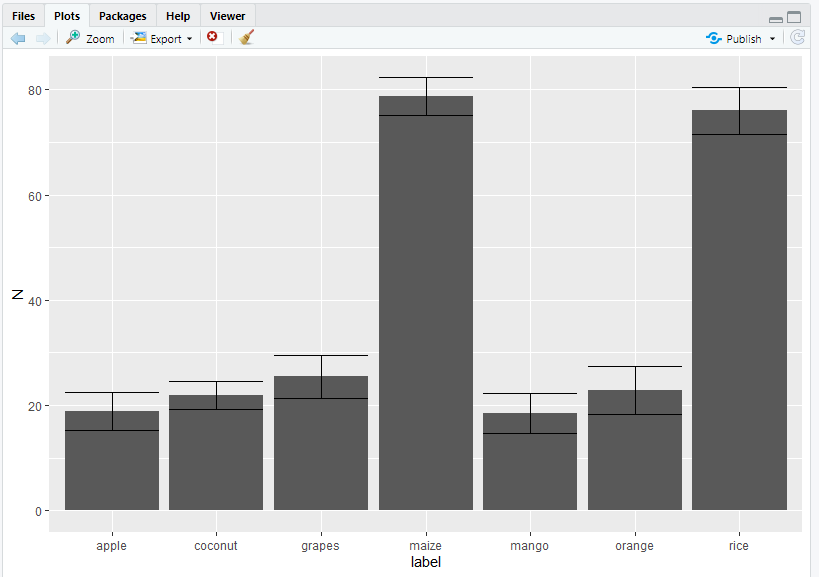My Personal Notes arrow_drop_up